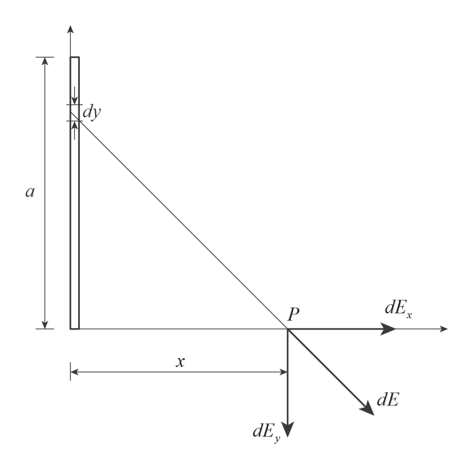Positive charge Q is distributed uniformly along the positive y-axis between y=0 and y=a. A negative point charge −q lies on the positive x-axis, a distance x from the originCalculate the x-component of the electric field produced by the charge distribution Q at points on the positive x-axis.Express your answer in terms of the variables Q, x, y, a and appropriate constants. Calculate the y-component of the electric field produced by the charge distribution Q at points on the positive x-axis.Express your answer in terms of the variables Q, x, y, a and appropriate constants. Calculate the x-component of the force that the charge distribution Q exerts on q.Express your answer in terms of the variables Q, q, x, y, a and appropriate constants. Calculate the y-component of the force that the charge distribution Q exerts on q.Express your answer in terms of the variables Q, q, x, y, a and appropriate constants.

Question

Positive charge Q is distributed uniformly along the positive y-axis between y=0 and y=a. A negative point charge −q lies on the positive x-axis, a distance x from the origin

Calculate the x-component of the electric field produced by the charge distribution Q at points on the positive x-axis.
Express your answer in terms of the variables Q, x, y, a and appropriate constants.

Calculate the y-component of the electric field produced by the charge distribution Q at points on the positive x-axis.
Express your answer in terms of the variables Q, x, y, a and appropriate constants.

Calculate the x-component of the force that the charge distribution Q exerts on q.
Express your answer in terms of the variables Q, q, x, y, a and appropriate constants.

Calculate the y-component of the force that the charge distribution Q exerts on q.
Express your answer in terms of the variables Q, q, x, y, a and appropriate constants.
Step 1

The uniform charge distribution Q is along the positive y axis.help_outlineImage Transcriptionclosedy а dE Р "х х dE dE fullscreen
Step 2

The charge Q is uniformly distributed along the axis y = 0 to y = a. The linear charge density of the charge distribution is

Step 3

The charge across the s...

Want to see the full answer?

See Solution

Want to see this answer and more?

Our solutions are written by experts, many with advanced degrees, and available 24/7

See Solution
Tagged in

Science